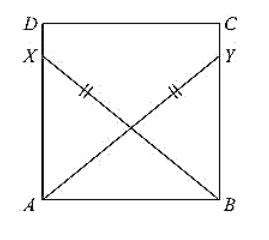# ABCD is a square, X and Y are points on sides AD and BC respectively such that

Question:

ABCD is a square, X and Y are points on sides AD and BC respectively such that AY = BX. Prove that BY = AX and ∠BAY = ∠ABX.

Solution:

Given that ABCD is a square, X and Y are points on sides AD and BC respectively such that AY = BX.

To prove BY = AX and ∠BAY = ∠ABX

Join B and X, A and Y.

Since, ABCD is a square

∠DAB = ∠CBA = 90°

∠XAB = ∠YAB = 90° .... (i)

Now, consider triangle XAB and YBA

We have

∠XAB = ∠YBA = 90°. [From (i)] BX = AY [given]

And AB = BA [Common side]

So, by RHS congruence criterion, we have ΔXAB ≅ ΔYBA

Now, we know that corresponding parts of congruent triangles are equal.

BY = AX and ∠BAY = ∠ABX

Hence proved